# Fleet Data Analysis

 Chapter 6.3: Fleet Data Analysis

 Chapter 6.3 Fleet Data Analysis

## Contents

Available Software:
RGA

More Resources:
RGA Examples Collection

Fleet analysis is similar to the repairable systems analysis described in the previous chapter. The main difference is that a fleet of systems is considered and the models are applied to the fleet failures rather than to the system failures. In other words, repairable system analysis models the number of system failures versus system time, whereas fleet analysis models the number of fleet failures versus fleet time.

The main motivation for fleet analysis is to enable the application of the Crow Extended model for fielded data. In many cases, reliability improvements might be necessary on systems that are already in the field. These types of reliability improvements are essentially delayed fixes (BD modes) as described in the Crow Extended chapter.

## Introduction

Recall from the previous chapter that in order to make projections using the Crow Extended model, the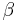of the combined A and BD modes should be equal to 1. Since the failure intensity in a fielded system might be changing over time (e.g., increasing if the system wears out), this assumption might be violated. In such a scenario, the Crow Extended model cannot be used. However, if a fleet of systems is considered and the number of fleet failures versus fleet time is modeled, the failures might become random. This is because there is a mixture of systems within a fleet, new and old, and when the failures of this mixture of systems are viewed from a cumulative fleet time point of view, they may be random. The next two figures illustrate this concept. The first picture shows the number of failures over system age. It can be clearly seen that as the systems age, the intensity of the failures increases (wearout). The superposition system line, which brings the failures from the different systems under a single timeline, also illustrates this observation. On the other hand, if you take the same four systems and combine their failures from a fleet perspective, and consider fleet failures over cumulative fleet hours, then the failures seem to be random. The second picture illustrates this concept in the System Operation plot when you consider the Cum. Time Line. In this case, theof the fleet will be equal to 1 and the Crow Extended model can be used for quantifying the effects of future reliability improvements on the fleet.

## Methodology

The figures above illustrate that the difference between repairable system data analysis and fleet analysis is the way that the data set is treated. In fleet analysis, the time-to-failure data from each system is stacked to a cumulative timeline. For example, consider the two systems in the following table.

System Failure Times (hr) End Time (hr) System Data 1 3, 7 10 2 4, 9, 13 15

### Convert to Accumulated Timeline

The data set is first converted to an accumulated timeline, as follows:

• System 1 is considered first. The accumulated timeline is therefore 3 and 7 hours.
• System 1's end time is 10 hours. System 2's first failure is at 4 hours. This failure time is added to System 1's end time to give an accumulated failure time of 14 hours.
• The second failure for System 2 occurred 5 hours after the first failure. This time interval is added to the accumulated timeline to give 19 hours.
• The third failure for System 2 occurred 4 hours after the second failure. The accumulated failure time is 19 + 4 = 23 hours.
• System 2's end time is 15 hours, or 2 hours after the last failure. The total accumulated operating time for the fleet is 25 hours (23 + 2 = 25).

In general, the accumulated operating time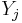is calculated by: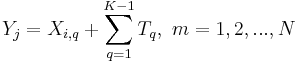where:

•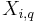is the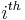failure of the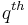system
•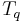is the end time of thesystem
•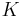is the total number of systems
•is the total number of failures from all systems (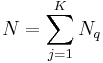)

As this example demonstrates, the accumulated timeline is determined based on the order of the systems. So if you consider the data in the table by taking System 2 first, the accumulated timeline would be: 4, 9, 13, 18, 22, with an end time of 25. Therefore, the order in which the systems are considered is somewhat important. However, in the next step of the analysis, the data from the accumulated timeline will be grouped into time intervals, effectively eliminating the importance of the order of the systems. Keep in mind that this will NOT always be true. This is true only when the order of the systems was random to begin with. If there is some logic/pattern in the order of the systems, then it will remain even if the cumulative timeline is converted to grouped data. For example, consider a system that wears out with age. This means that more failures will be observed as this system ages and these failures will occur more frequently. Within a fleet of such systems, there will be new and old systems in operation. If the data set collected is considered from the newest to the oldest system, then even if the data points are grouped, the pattern of fewer failures at the beginning and more failures at later time intervals will still be present. If the objective of the analysis is to determine the difference between newer and older systems, then that order for the data will be acceptable. However, if the objective of the analysis is to determine the reliability of the fleet, then the systems should be randomly ordered.

### Analyze the Grouped Data

Once the accumulated timeline has been generated, it is then converted into grouped data. To accomplish this, a group interval is required. The group interval length should be chosen so that it is representative of the data. Also note that the intervals do not have to be of equal length. Once the data points have been grouped, the parameters can be obtained using maximum likelihood estimation as described in the Crow-AMSAA (NHPP) chapter. The data from the table above can be grouped into 5 hour intervals. This interval length is sufficiently large to insure that there are failures within each interval. The grouped data set is given in the following table.

Failures in Interval Interval End Time Grouped Data 1 5 1 10 1 15 1 20 1 25

The Crow-AMSAA model for grouped failure times is used for the data, and the parameters of the model are solved by satisfying the following maximum likelihood equations (See Crow-AMSAA (NHPP)):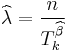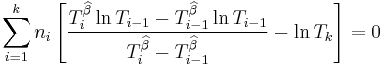## Fleet Analysis Example

The following table presents data for a fleet of 27 systems. A cycle is a complete history from overhaul to overhaul. The failure history for the last completed cycle for each system is recorded. This is a random sample of data from the fleet. These systems are in the order in which they were selected. Suppose the intervals to group the current data are 10,000; 20,000; 30,000; 40,000 and the final interval is defined by the termination time. Conduct the fleet analysis.

System Cycle Time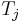Number of failures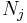Failure Time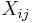Sample Fleet Data 1 1396 1 1396 2 4497 1 4497 3 525 1 525 4 1232 1 1232 5 227 1 227 6 135 1 135 7 19 1 19 8 812 1 812 9 2024 1 2024 10 943 2 316, 943 11 60 1 60 12 4234 2 4233, 4234 13 2527 2 1877, 2527 14 2105 2 2074, 2105 15 5079 1 5079 16 577 2 546, 577 17 4085 2 453, 4085 18 1023 1 1023 19 161 1 161 20 4767 2 36, 4767 21 6228 3 3795, 4375, 6228 22 68 1 68 23 1830 1 1830 24 1241 1 1241 25 2573 2 871, 2573 26 3556 1 3556 27 186 1 186 Total 52110 37

Solution

The sample fleet data set can be grouped into 10,000; 20,000; 30,000; 40,000 and 52,110 time intervals. The following table gives the grouped data.

Time Observed Failures Grouped Data 10,000 8 20,000 16 30,000 22 40,000 27 52,110 37

Based on the above time intervals, the maximum likelihood estimates of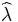and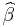for this data set are then given by: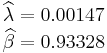The next figure shows the System Operation plot.

## Applying the Crow Extended Model to Fleet Data

As it was mentioned previously, the main motivation of the fleet analysis is to apply the Crow Extended model for in-service reliability improvements. The methodology to be used is identical to the application of the Crow Extended model for Grouped Data described in a previous chapter. Consider the fleet data from the example above. In order to apply the Crow Extended model, put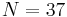failure times on a cumulative time scale over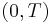, where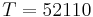. In the example, each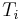corresponds to a failure time. This is often not the situation. However, in all cases the accumulated operating timeat a failure time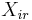is: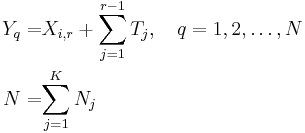And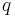indexes the successive order of the failures. Thus, in this example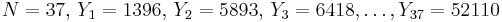. See the table below.ModeTest-Find-Test Fleet Data 1 1396 BD1 20 26361 BD1 2 5893 BD2 21 26392 A 3 6418 A 22 26845 BD8 4 7650 BD3 23 30477 BD1 5 7877 BD4 24 31500 A 6 8012 BD2 25 31661 BD3 7 8031 BD2 26 31697 BD2 8 8843 BD1 27 36428 BD1 9 10867 BD1 28 40223 BD1 10 11183 BD5 29 40803 BD9 11 11810 A 30 42656 BD1 12 11870 BD1 31 42724 BD10 13 16139 BD2 32 44554 BD1 14 16104 BD6 33 45795 BD11 15 18178 BD7 34 46666 BD12 16 18677 BD2 35 48368 BD1 17 20751 BD4 36 51924 BD13 18 20772 BD2 37 52110 BD2 19 25815 BD1

Each system failure time in the table above corresponds to a problem and a cause (failure mode). The management strategy can be to not fix the failure mode (A mode) or to fix the failure mode with a delayed corrective action (BD mode). There are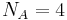failures due to A failure modes. There are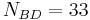total failures due to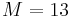distinct BD failure modes. Some of the distinct BD modes had repeats of the same problem. For example, mode BD1 had 12 occurrences of the same problem. Therefore, in this example, there are 13 distinct corrective actions corresponding to 13 distinct BD failure modes.

The objective of the Crow Extended model is to estimate the impact of the 13 distinct corrective actions.The analyst will choose an average effectiveness factor (EF) based on the proposed corrective actions and historical experience. Historical industry and government data supports a typical average effectiveness factor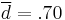for many systems. In this example, an average EF of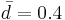was assumed in order to be conservative regarding the impact of the proposed corrective actions. Since there are no BC failure modes (corrective actions applied during the test), the projected failure intensity is: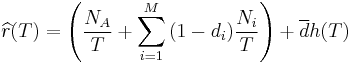The first term is estimated by: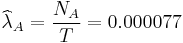The second term is: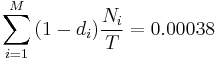This estimates the growth potential failure intensity: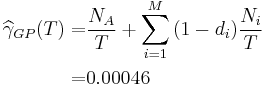To estimate the last term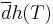of the Crow Extended model, partition the data in the table into intervals. This partition consists of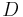successive intervals. The length of theinterval is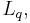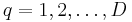. It is not required that the intervals be of the same length, but there should be several (e.g., at least 5) cycles per interval on average. Also, let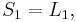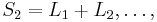etc. be the accumulated time through theinterval. For theinterval, note the number of distinct BD modes,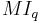, appearing for the first time,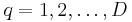. See the following table.

Interval No. of Distinct BD Mode Failures Length Accumulated Time Grouped Data for Distinct BD Modes 1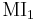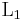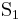2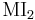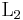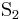. . . . . . . . . . . . D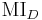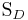The term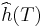is calculated as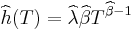and the valuesandsatisfy the maximum likelihood equations for grouped data (given in the Methodology section). This is the grouped data version of the Crow-AMSAA model applied only to the first occurrence of distinct BD modes.

For the data in the first table, the first 4 intervals had a length of 10,000 and the last interval was 12,110. Therefore,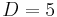. This choice gives an average of about 5 overhaul cycles per interval. See the table below.

Interval No. of Distinct BD Mode Failures Length Accumulated Time Grouped Data for Distinct BD Modes from Data in "Applying the Crow Extended Model to Fleet Data" 1 4 10000 10000 2 3 10000 20000 3 1 10000 30000 4 0 10000 40000 5 5 12110 52110 Total 13

Thus: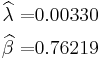This gives: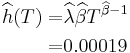Consequently, for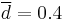the last term of the Crow Extended model is given by: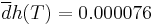The projected failure intensity is: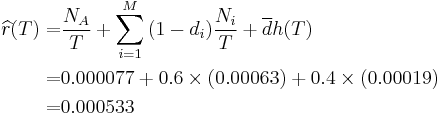This estimates that the 13 proposed corrective actions will reduce the number of failures per cycle of operation hours from the currentto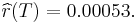The average time between failures is estimated to increase from the current 1408.38 hours to 1876.93 hours.

## Confidence Bounds

For fleet data analysis using the Crow-AMSAA model, the confidence bounds are calculated using the same procedure described for the Crow-AMSAA (NHPP) model (See Crow-AMSAA Confidence Bounds). For fleet data analysis using the Crow Extended model, the confidence bounds are calculated using the same procedure described for the Crow Extended model (See Crow Extended Confidence Bounds).

# More Examples

## Predicting the Number of Failures for Fleet Operation

11 systems from the field were chosen for fleet analysis. Each system had at least one failure. All of the systems had a start time equal to zero and the last failure for each system corresponds to the end time. Group the data based on a fixed interval of 3,000 hours, and assume a fixed effectiveness factor equal to 0.4. Do the following:

1. Estimate the parameters of the Crow Extended model.
2. Based on the analysis, does it appear that the systems were randomly ordered?
3. After the implementation of the delayed fixes, how many failures would you expect within the next 4,000 hours of fleet operation.
System Times-to-Failure Fleet Data 1 1137 BD1, 1268 BD2 2 682 BD3, 744 A, 1336 BD1 3 95 BD1, 1593 BD3 4 1421 A 5 1091 A, 1574 BD2 6 1415 BD4 7 598 BD4, 1290 BD1 8 1556 BD5 9 55 BD4 10 730 BD1, 1124 BD3 11 1400 BD4, 1568 A

Solution

1. The next figure shows the estimated Crow Extended parameters.
2. Upon observing the estimated parameter, it does appear that the systems were randomly ordered since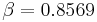. This value is close to 1. You can also verify that the confidence bounds oninclude 1 by going to the QCP and calculating the parameter bounds or by viewing the Beta Bounds plot. However, you can also determine graphically if the systems were randomly ordered by using the System Operation plot as shown below. Looking at the Cum. Time Line, it does not appear that the failures have a trend associated with them. Therefore, the systems can be assumed to be randomly ordered.
3. After implementing the delayed fixes, the system's projected MTBF is equal toas shown in the plot below.

To estimate the number of failures during the next 4,000 hours, calculate the following: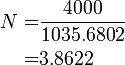Therefore, it is estimated that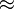4 failures will be observed during the next 4,000 hours of fleet operation.